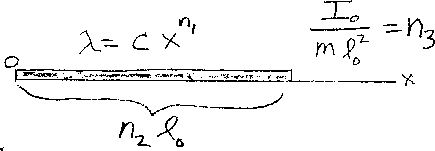Problem D6: A thin rod has a non-uniform linear mass density. If the left end is at x=0, the linear mass density is λ = c xn1 where c is a constant. The length of the rod is n2 l0. Find the ratio of the rotational inertial I about the left end to l02. If I/(ml02) = n3, what is n3? Note: n1, n2, and n3 are unitless.n1 = n2 = Input n3:
If you are currently in my class, you can record your grade by entering your name and student ID number (without the leading zeros) below and clicking on "record grade".
 First Name = Last Name = ID = Problem: